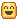# Pacejka car friction model makes the car jitter and i don't know why

hello, so recently I have developed a car chassis using a raycast suspension and the Pacejka friction model. But there is an issue, the car vibrates constantly at high/low speeds here is how I implemented it

``````function pacejka(slipAngleDegrees)
local x = slipAngleDegrees
local B = 1
local C = 1
local D = 1.3
local E = -1

return D * math.sin(C * math.atan(B * x - E * (B * x - math.atan(B * x))))
end

local multiplier = 60 / (1 / dt)

local tirePushingGroundValue=springLenghts[i]/restSpringLenght-- the problem isn't here
local tireVelocity = carCenterMassPart:GetVelocityAtPosition(rayResult.Position)
local cf=carCenterMassPart.CFrame

if i==1 or i==2 then
end

local rv= cf.RightVector
local lv= cf.LookVector

local lateralVelocity = tireVelocity:Dot(rv)
local longitudinalVelocity = tireVelocity:Dot(lv)

local slipAngleDeg = math.deg(math.atan2(lateralVelocity,longitudinalVelocity))
local pacejkaValue = pacejka(slipAngleDeg)

local lateralForce = (tireLoad * pacejkaValue) / 4
carCenterMassPart:ApplyImpulseAtPosition(multiplier*(-1*carCenterMassPart.CFrame.RightVector * (lateralForce)),rayResult.Position)-- the issue is probably here but don't know any other way to apply the force in an responsive way
``````

code is taken from here
the result: https://gyazo.com/bb507e3c6aeda4f44301475ba983a93a

thank you for your timefixed by implementing a lateral friction clamp (can be found here also it has some amazing vehicle handling concepts that you can learn from too)

``````local lowerValue = (tireLoad*lateralVelocity*lateralVelocity)*dt